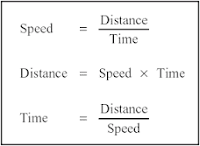### Speed, Distance and Time- Important Formulae, Tricks and Shortcuts [Quantitative Aptitude]

Here are important formulas and shortcuts to solve problems related to Speed, Distance and Time. Basic knowledge regarding calculating speed, distance and time is important for various competitive exams like IBPS, SBI Bank PO, assistant, SSC exams, Railway exam, UPSC exams.### What is Speed?

Let a moving car covers 40 Kms in 1 Hour so we can say that car is moving at speed of 40Km/hr or a bus covers 70 Km in 1 hour so speed of bus is 70 Km/hr.

So speed is distance traveled per unit time.

### I. From km/hr to m/sec

1 km = 1000 meter. 1 hr = 3600 seconds.

=> 1 km/hr = (1000/3600) m/sec = (5/18) m/sec

### => x km/hr = x * (5/18)  m/sec

Eg 1: Convert 72 km/hr in m/sec

Soln: 72 km/hr = 72 * (5/18) m/sec = 20 m/sec

Eg 2:  Covert 45 km/hr in m/sec

Soln: 45 km/hr = 45 * (5/18) m/sec = 12.5 m/sec.

### II. From m/sec to km/hr.

1m = 1/1000 km, 1 sec = 1/3600 hr.

1 m/s = (3600/1000) km/hr = (18/5) km/hr

### => x m/s = x * (18/5) km/hr.

Eg 1 : Convert 30 m/sec in km/hr

Soln: 30 m/sec = 30 * (18/5) km/hr = 108 km/hr.

Eg 2: Convert 15 m/sec in km/hr

Soln: 15 m/sec = 15 * (18/5) km/hr = 54 km/hr.

### Trick 1: If ratio of speed of two objects is A:B then the time taken by them to cover the same distance is B:A.

Eg 1: Two cars are moving with speed of 45 km/hr and 60 km/hr. Find the ratio of time taken by them to cover 20 Kms.

Soln: Ratio of speeds of cars is 45 : 60 = 3 : 4
Hence ration of time taken is  4 : 3.

### Trick 2 (I): If an object covers certain distance with x km/hr speed and equal distance at y km/hr. Then, the average speed during journey is {2xy/(x+y)} km/hr.

Eg: A man covers 10 kms by running at speed of 15 km/hr. Next 10 kms he cover by car at speed of 45 km/hr. Find his average speed during whole journey.

Soln: Avg speed =( 2 * 15 * 45)  / (15 + 45) =  22.5 Km/hr

### Trick 2 (II) : If an object covers d1 distance with x km/hr speed and d2 distance at y km/hr. Then, the average speed during journey is {(d1+d2)xy/(d2x+d1y)} km/hr.

Eg: A man covers 10 kms by running at speed of 15 km/hr. Next 20 kms he cover by car at speed of 45 km/hr. Find his average speed during whole journey in m/sec.

Soln: Avg speed = {(10 + 20) * 15 * 45}  / (20*15 + 10*45) =  27 Km/hr
= 27 * (5/18) m/sec = 7.5 m/sec.

## Want to Stay in Contact !

Get new posts and updates in your inbox. Subscribe through our Feedburner Email subscription.